# What is Subtraction with Regrouping?

Instructor: T.J. Hoogsteen

T.J. is currently a grade 5 teacher and Vice-Principal. He has a master's degree in Educational Administration and is working toward an Ed.D. in Educational Leadership.

In this lesson you will learn what regrouping means, why it is important, when it is needed in subtraction, and how to do it. You will get a chance to practice regrouping during the quiz.

## What Is Regrouping?

Everyone at one time or another has played a game with a group of friends when all of a sudden another friend comes along and wants to join in. This leaves the group with a dilemma: how do they include their friend and still make sure that the teams are evenly matched? One choice the friends can make is to regroup; that is, to borrow or move a friend or two to another team to make sure that each team has enough to play.

That is much like regrouping in subtraction, which is when we borrow from one group and give to another so that the operation can be completed. Regrouping doesn't always need to be done in every subtraction problem, but when it is necessary it's a very important step that, if skipped, can mess up a calculation.

## When Is Regrouping Required?

So when exactly do you need to regroup? Here's an example of a two digit subtraction equation that needs regrouping, written vertically: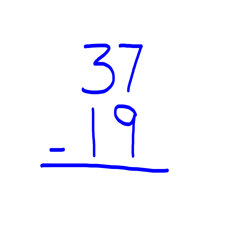As with other basic operation equations, you always start working in the far right column - the ones column - and subtract the bottom digit from the top digit. In most subtraction situations, you can't subtract a bigger number from a smaller number. But here, you will notice the top digit (7) is smaller than the bottom digit (9).

When the top digit is less than the bottom digit, you need regrouping. To regroup, you borrow one ten from the tens column and add it to the ones column.

## How to Regroup

Now I'll demonstrate two different ways to represent regrouping. First, with base ten blocks.

Here's 37: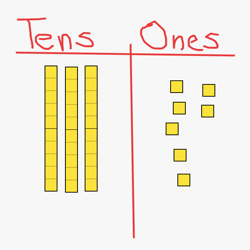When I try to take away 9 ones, I notice I only have 7 so I can't. So I borrow a 10 from the tens column.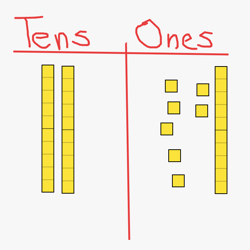Now I have 17 ones.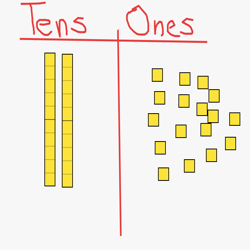And I can take away 9.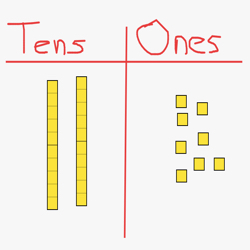Then I'm left with 8.

Here is what the same process looks like when it is written:I can't subtract 9 from 7, so I regroup and borrow a 10 (I do this by crossing out the 3, subtracting 1 and making it 2, and adding a 10 to the ones column).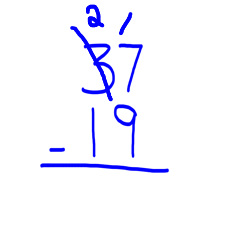Now I have 17 ones and I can subtract 9.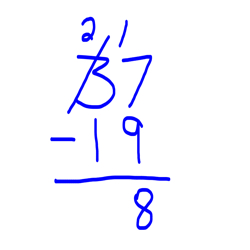I'm left with 8 ones.

Now to finish my equation, I just subtract the 2 digit in the tens column (2-1).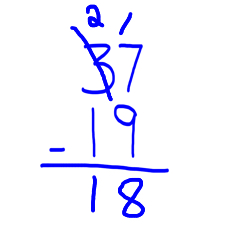My solution is 18.

To unlock this lesson you must be a Study.com Member.

### Register to view this lesson

Are you a student or a teacher?

#### See for yourself why 30 million people use Study.com

##### Become a Study.com member and start learning now.
Back
What teachers are saying about Study.com

### Earning College Credit

Did you know… We have over 200 college courses that prepare you to earn credit by exam that is accepted by over 1,500 colleges and universities. You can test out of the first two years of college and save thousands off your degree. Anyone can earn credit-by-exam regardless of age or education level.